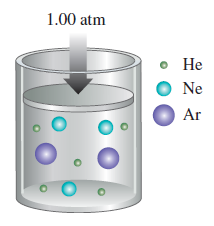# Problem: Determine the partial pressure of each gas as shown in the figure below. Note: The relative numbers of each type of gas are depicted in the figure.

🤓 Based on our data, we think this question is relevant for Professor McCarren's class at UIUC.

###### FREE Expert Solution

Recall that the partial pressure of a gas (PGas) in a mixture is given by:

$\overline{){{\mathbf{P}}}_{{\mathbf{gas}}}{\mathbf{=}}{{\mathbf{\chi }}}_{{\mathbf{gas}}}{{\mathbf{P}}}_{{\mathbf{total}}}}$

Mole fraction:

Solving for partial pressure of each gas:

• # of particles = # of moles of each gas

PHe0.50 atm###### Problem Details

Determine the partial pressure of each gas as shown in the figure below. Note: The relative numbers of each type of gas are depicted in the figure.